# Fraction Worksheets

#### What are Fractions?

When you have a value that is less than one whole unit, we have a number of ways to describe that value. One method of doing this is to use fractions. A fraction is a way to describe how many parts of the whole that we have present. In mathematics, there is vast variety when it comes to types of numbers. These numbers can either be whole numbers or fractions. All numbers; -infinite, -223 1, 2, 3, 4, 5, … , 332211, 332212, ... , +infinite, all whole numbers. When we take a portion of these whole numbers, we call them fractions. This number is broken into two portions. The top half or starting part of the fraction is called the numerator it tells us how many parts of the whole we possess. The bottom or last number is called the denominator and it tells us how many parts there are in one complete unit. A common way to understand this is to consider the concept of a six-pack of soda. If you have removed and used three of the six cans, we can describe this with the fraction 3/6. Fractions can also be reduced to express a concept and all we have to do is find a number or divisor that can be applied to both the numerator and denominator. In the case of six-pack of soda, 3 goes into both the numerator and denominator. We can reduce that value to 1/2 or half. There are times that the numerator can be larger than the denominator we refer to this as improper fractions. In those cases, we can form mixed numbers which are a mix of whole numbers and fractions. There are two types of fractions, proper fractions, and improper fractions. Proper fractions are ones where the denominator is greater than the numerator. The value of these fractions lies between 0 and 1. Improper fractions are those where the numerator is greater than the denominator. The value of these is greater than 1.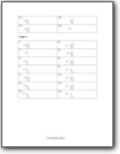Students will learn how to add fractions and mixed numbers.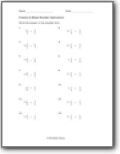###### Fraction Subtraction

Students will learn how to find the difference between fractions and mixed numbers.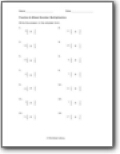###### Fraction Multiplication

Learn how multiply fractions and mixed numbers.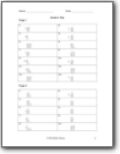###### Fraction Division

We will work on processing quotients between fractions and mixed number sets.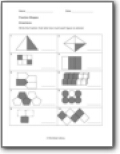###### Fraction Shapes

Write the fraction that tells how much each figure is colored.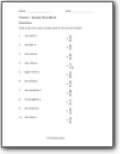###### Number Word Match

List the factors for each number. List the common factors and greatest common factor of each pair of numbers.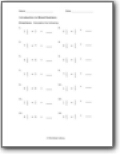###### Introduction to Mixed Numbers

Learn to breakdown mixed numbers.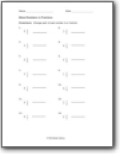###### Mixed Numbers to Fractions

Change each mixed number to a fraction.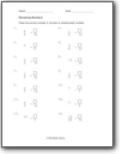###### Renaming Numbers

Place the correct number in the box to rename each number.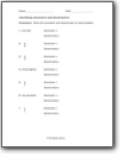###### Number Words, Breaking Numerators and Denominators

Write the numerator and denominator for each problem.###### Fractions from Number Words

Place the correct number in the box to rename each number.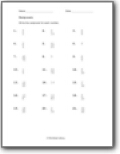###### Reciprocals

Write the reciprocal for each number.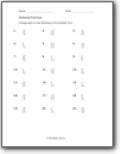###### Reducing Fractions

Change each of the following to the simplest form.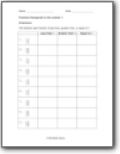###### Fractions Compared to the number 1

Tell whether each fraction is less than, greater than, or equal to 1.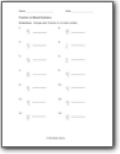###### Fraction to Mixed Numbers

Change each fraction to a mixed number.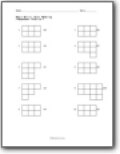###### Color Modeling

You will be given a fraction that use must model within a shape by coloring it.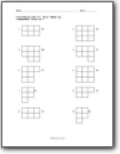###### Color Modeling Intermediate Skills

You will be given a fraction that use must model within a shape by coloring it.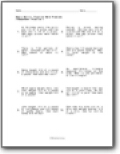###### Basic Skills: Fraction Word Problems

This is the start of your introduction to word problems with more simple values to work with.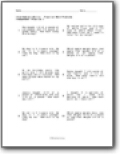###### Intermediate Skills: Fraction Word Problems

We work on a bit more complex problem set. Here is an example: My recipe calls for 2/3 cups of white flour and 2 1/5 cups of whole wheat flour. How much flour do I need in total for my recipe?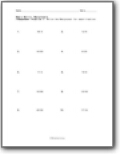###### Basic and Intermediate Skills: Reciprocals

Write the reciprocal for each fraction.

### How Fractions Are Used in the Real World?

Fractions are a concept which we use daily. However, we do not realize that we are using them. For instance, you are on a shopping spree with your birthday money of \$100, and you have different items on your list. And then, you see that there 75% discount on your favorite store. As much as the good news is, you still need to do some math. In other words, you must multiply the item by 3/4 to attain the discounted price.